R语言中的颜色以及色板

### 1.R语言中的默认颜色

R中可以通过定义col参数自定义颜色。有以下四种方法进行设置，是等价的：

1. 数字：（如1代表当前palette的第1种颜色，2代表当前palette的第2种颜色等）；
2. 颜色名：（如”red”,”blue”)、可以使用colors()获得所有颜色名称列表
3. RGB：使用rgb()函数得到的返回值 rgb(1, 0, 0)
4. 十六进制颜色代码：#FF0000

`barplot(1:4, col = c(2, "red", rgb(1, 0, 0), "#FF0000"))`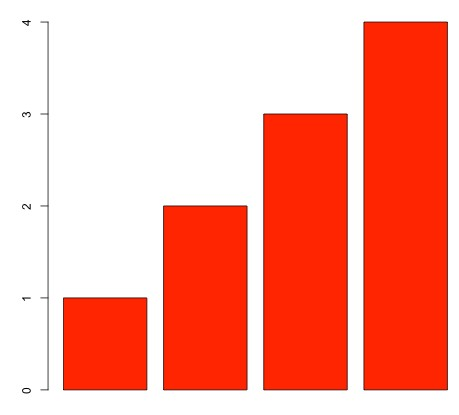```palette()

##  "black"   "red"     "green3"  "blue"    "cyan"    "magenta" "yellow" ##  "gray"```

```palette(rainbow(12))
barplot(1:4, col = c(2, "red", rgb(1, 0, 0), "#FF0000"))```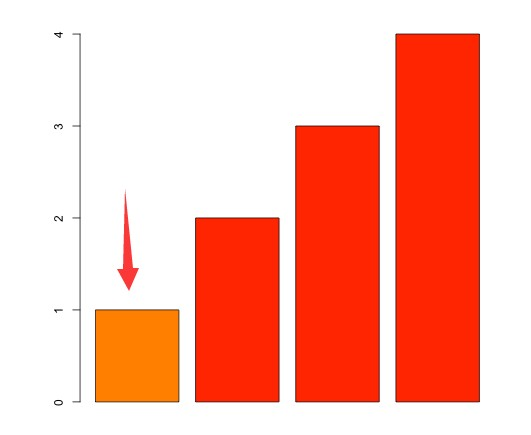`palette("default")`

### 2.R预置色彩系统的色板

R语言的预设调色板一共有五个：

```rainbow
heat.colors
terrain.colors
topo.colors
cm.colors```

```rainbow(10)
 "#FF0000FF" "#FF9900FF" "#CCFF00FF" "#33FF00FF" "#00FF66FF" "#00FFFFFF"
 "#0066FFFF" "#3300FFFF" "#CC00FFFF" "#FF0099FF"```

```library(scales)
show_col(rainbow(10),labels=T) #labels控制是否显示HEX格式的色值信息.```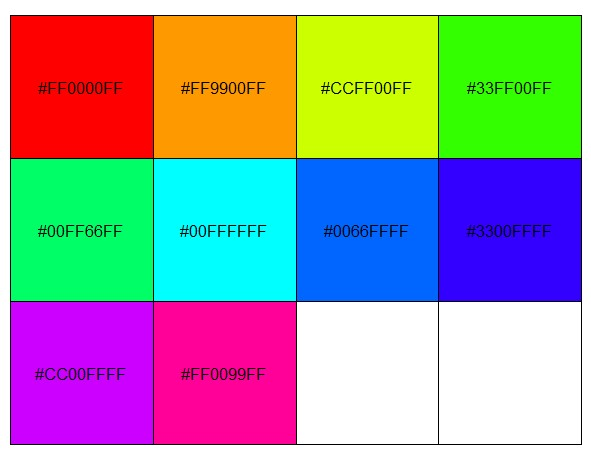```par(mfrow=c(1,5),mar=c(0.5,0.5,2,0.5),xaxs="i",yaxs="i")
n<-1000
barplot(rep(1,times=n),col=rainbow(n),border=rainbow(n),horiz=T,axes=F,main="Rainbow Color")
barplot(rep(1,times=n),col=heat.colors(n),border=heat.colors(n),horiz=T,axes=F,main="Heat.Colors")
barplot(rep(1,times=n),col=terrain.colors(n),border=terrain.colors(n),horiz=T,axes=F,main="Terrain.Colors")
barplot(rep(1,times=n),col=topo.colors(n),border=topo.colors(n),horiz=T,axes=F,main="Topo.Colors")
barplot(rep(1,times=n),col=cm.colors(n),border=cm.colors(n),horiz=T,axes=F,main="Cm.Colors")```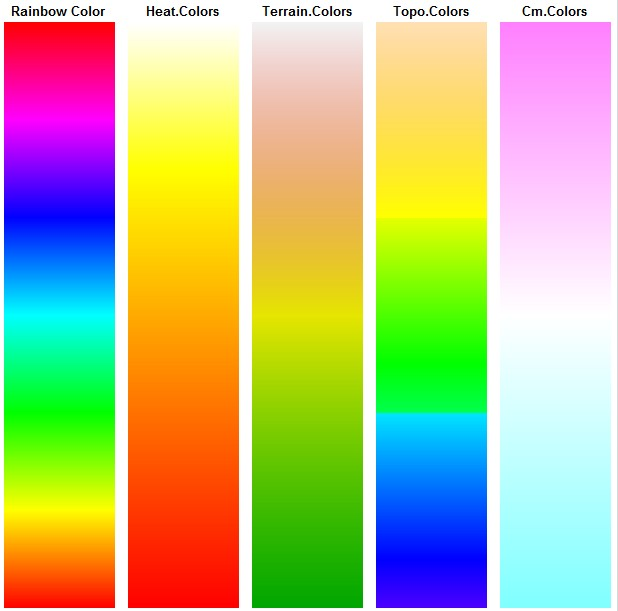```dev.off()#关闭上次的绘图面板
par(mfrow=c(2,1),mar=c(0.5,0.5,2,0.5),xaxs="i",yaxs="i")
a<-heat.colors(10)
b<-topo.colors(10)
barplot(rep(1,times=10),col=b,border=b,main="Topo.Colors10",axes=F)
barplot(rep(1,times=10),col=a,border=a,main="Heat.Colors10",axes=F)```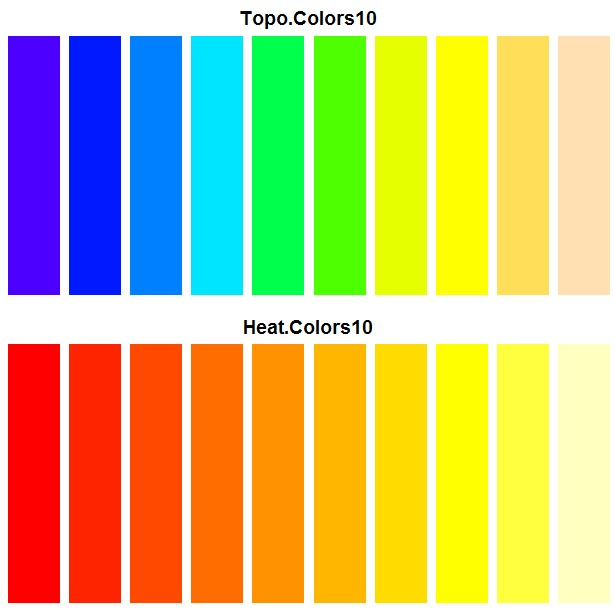```dev.off()
c<-c(a[c(1,3,5,7,9)],b[c(2,4,6,8,10)])
barplot(rep(1,times=10),col=c,border=c,axes=F,main="topo&heat 10")```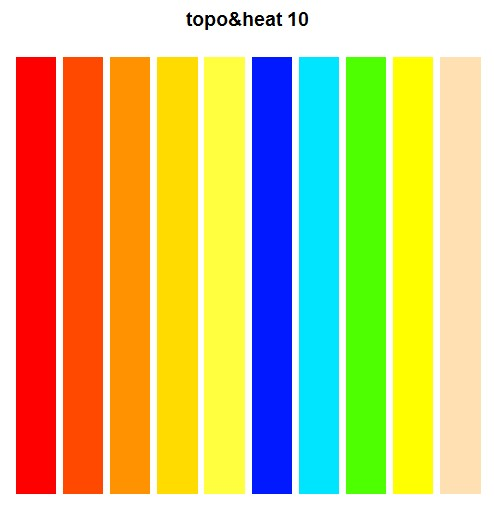### 3.产生渐变颜色colorRamp()和colorRampPalette()：

colorRamp()和colorRampPalette()都可用于建立颜色板。想象一下你现在有一个色板，色板上有红色，蓝色，和白色，你就可以用colorRamp()和colorRampPalette()基于这三个颜色就行调色,两者的不同在于，colorRamp() 可以指定一个小数，返回一个指定的颜色值，需要用rgb方法转换；

colorRampPalette()返回指定数量的颜色值，两个方法可以达到相同的效果：

```par(mfrow = c(1, 2))
b2p1 <- colorRampPalette(c("red", "white","blue"))
b2p2 <- colorRamp(c("red", "white","blue"))

barplot(rep(1, 12), axes=F, col = b2p1(12), border = b2p1(12), main = "colorRampPalette")

rgb( b2p2(seq(0, 1, length = 12)), max = 255)

barplot(rep(1, 12), axes=F,col = rgb( b2p2(seq(0, 1, length = 12)), max = 255), border = rgb( b2p2(seq(0, 1, length = 12)), max = 255), main = "colorRamp")
```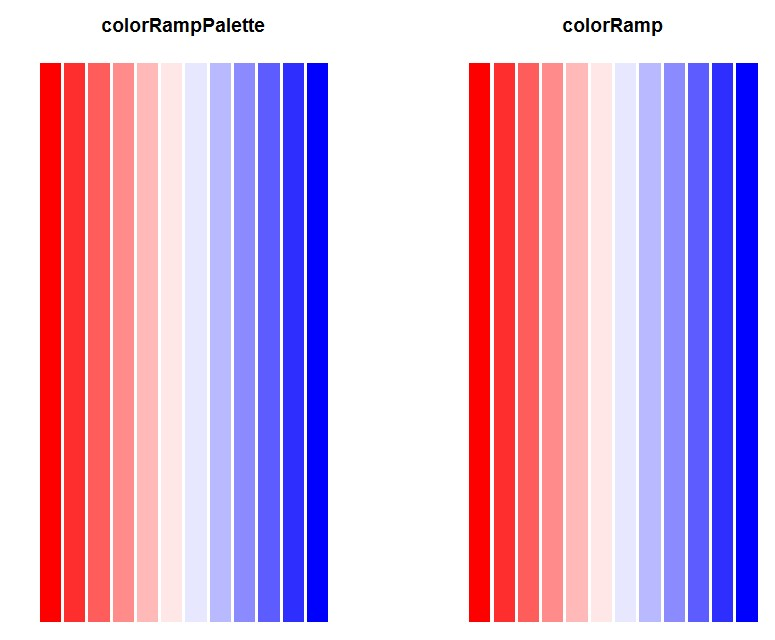### 4.RColorBrewer包色板

`require("RColorBrewer")display.brewer.all()`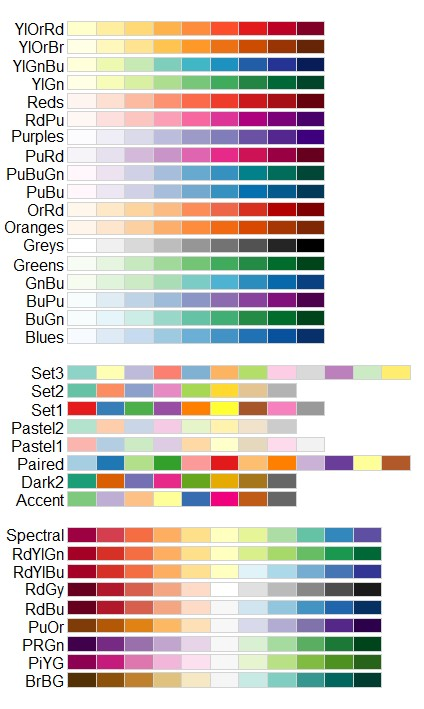取出对应色板颜色，以及数量：

`brewer.pal(9, "Purples") "#FCFBFD" "#EFEDF5" "#DADAEB" "#BCBDDC" "#9E9AC8" "#807DBA" "#6A51A3" "#54278F" "#3F007D"`

• 发表于 2019-03-13 15:51
• 阅读 ( 3911 )
• 分类：R

## 作家榜 »

1.omicsgene 288 文章
2.安生水 215 文章
3.Daitoue 168 文章
4.生物女学霸 120 文章
5.红橙子 37 文章
6.landy 37 文章
7.生信老顽童 35 文章
8.CORNERSTONE 32 文章